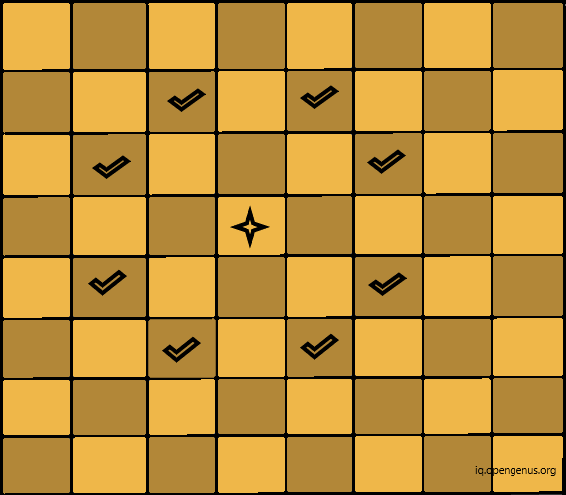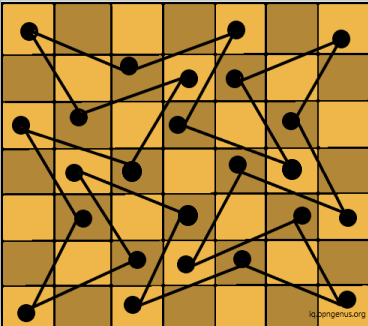×

Search anything:

# Knight’s Tour Problem

#### Algorithms backtrackingGet this book -> Problems on Array: For Interviews and Competitive Programming

The Knight's Tour Problem is one of the famous problem in which we have the knight on a chessboard. The knight is supposed to visit every square exactly once.

Following it we have two cases:

• Closed Tour : The knight ends on a square that is one move away from the beginning square. This means that if it continues to move, with the same path that it has followed till now, it will be able to traverse all squares again.
• Open Tour : The knight ends on any other square.
This problem is solved on a n x n chessboard.
"n" could have any value.
This problem is derived from the Hamiltonian Path Problem in Graph Theory.

Hamiltonian Path Problem is focused on finding out if there is a path or route in undirected graph from the beginning to ending node. However, it can gain a lot of complexity even on little increase in its size.
Hamiltonian Path is the one that visits each vertex of the graph only once. A grap h with Hamiltonian Path in it is called traceable graph.

However, there are ways to solve this problem in linear time.
Consider the below image, let the diamond represent the "knight" and the tick marks represent its possible moves at given position. A "knight" can move two squares in cardinal direction then one square in orthogonal direction.Example of Knight's Tour Problem for 7x7 board:There are many variants possible for this problem like having two knights and so on. In this article we will explore the basic problem with one knight and how it can cover all squares without revisiting them.

One way to solve this problem is Naive Approach, to find all possible configurations and choose the correct one. Since, this would take much longer if the value of n in n x n board is large.

Algorithm:

``````while all path are not visited
{
traverse next path
if all squares are covered {
print the path
}
}
``````

### Backtracking

Other technique that can be used for this problem is Backtracking. It is optimized version of our naive technique.
Backtracking is the technique in which we try all possible solutions or combinations till we find the one that is correct. In this each combination is tried only once.

It incrementally makes the solution using recursion. We consider on step at a time. If they do not satisfy the constraints then we remove those particular steps.

Algorithm:

``````If all squares have been visited then return the solution
Else:
Consider one move and check each move if it leads to solution recursively.
If it does not lead us to solution then remove it and check for another solution
If none of them works then:
If its not the first call, then return previous item to be added to solution
Else return "no solution exists"
``````

Time Complexity: O(n * n) for traversing the n x n squares.
Implementation:

``````bool range(int x, int y,int sol[n][n])
{
return ((x >= 0 && y >= 0) && (x < n && y < n)&&sol[x][y]==-1);
}
void print(int a[n][n])
{
for (int i = 0; i < n; ++i)
{
for (int j = 0; j < n; ++j)
printf("%d\t",a[x][y]);
printf("\n");
}
}
int aux(int x, int y, int move, int sol[n][n],int x[n], int y[n])
{
int k, next_x, next_y;
if (move == n * n)
return 1;

for (k = 0; k < 8; k++) {
next_x = x + x[k];
next_y = y + y[k];
if (range(next_x, next_y, sol)) {
sol[next_x][next_y] = move;
if (aux(next_x, next_y, move + 1, sol,x, y) == 1)
return 1;
else
// backtracking
sol[next_x][next_y] = -1;
}
}
return 0;
}

int ans()
{
int sol[n][n];

for (int x = 0; x < N; x++)
for (int y = 0; y < N; y++)
sol[x][y] = -1;
int x = { 2, 1, -1, -2, -2, -1, 1, 2 };
int y = { 1, 2, 2, 1, -1, -2, -2, -1 };
sol = 0;
if (aux(0, 0, 1, sol, x, y) == 0) {
cout << "No Solution";
return 0;
}
else
print(sol);

return 1;
}

``````

#### Explanation:

• The function called in main() would be ans().
• It declares a sol array representing the chess board of n x n size.
• Then we initialise it with -1 values. Then we define the next values of x and y coordinates in array x and array y.
• Now, we call function aux and explore all tours and check if the solution exists.
• If it exists then we print the solution.
The aux function:
• This is the recursive function to find the final solution.
• In this we try all possible moves from x and y. We also call function range to check if the squares are in n x n configuration.
• We essentially use backtracking to solve this problem.

We can have much better approaches as well to solve this problem:

## Warnsdorff’s algorithm:

In this approach we can start from any initial square on the chess board. Then, we will move to an adjacent, unvisited square with minimum unvisited adjacent squares.

Algorithm:

• Set a square as the initial position to start from.
• Mark it with current move number starting with "1".
• Make a set of all positions that can be visited from initial position.
• Mark the square out of the set of possible positions that can be visited with minimum accessibility from initial position as next move number.
• Following the above steps, return the marked board with move numbers with which it has been visited.
``````
bool range(int x, int y)
{
return ((x >= 0 && y >= 0) && (x < n && y < n));
}

bool isempty(int a[], int x, int y)
{
return (range(x, y)) && (a[y*n+x] < 0);
}
int degree(int a[], int x, int y)
{
int count = 0;
for (int i = 0; i < n; ++i)
if (isempty(a, (x + cx[i]), (y + cy[i])))
count++;

return count;
}

void print(int a[])
{
for (int i = 0; i < n; ++i)
{
for (int j = 0; j < n; ++j)
printf("%d\t",a[j*n+i]);
printf("\n");
}
}

bool neighbour(int x, int y, int xx, int yy)
{
for (int i = 0; i < n; ++i)
if (((x+cx[i]) == xx)&&((y + cy[i]) == yy))
return true;

return false;
}

bool next(int a[], int *x, int *y)
{
int min_degree_index = -1, c, min_degree = (n+1), nx, ny;
int start = rand()%n;
for (int count = 0; count < n; ++count)
{
int i = (start + count)%n;
nx = *x + cx[i];
ny = *y + cy[i];
if ((isempty(a, nx, ny))&&(c = degree(a, nx, ny)) < min_deg)
{
min_degree_index = i;
min_degree = c;
}
}
if (min_degree_index == -1)
return false;
nx = *x + cx[min_degree_index];
ny = *y + cy[min_degree_index];
a[ny*n + nx] = a[(*y)*n + (*x)]+1;
*x = nx;
*y = ny;

return true;
}

bool find()
{
int a[n*n]={-1};
int x1 = rand()%n;
int y1 = rand()%n;
int x = x1, y = y1;
a[y*n+x] = 1;
for (int i = 0; i < n*n-1; ++i)
if (next(a, &x, &y) == 0)
return false;
if (!neighbour(x, y, x1, y1))
return false;
print(a);
return true;
}
``````

#### Explanation:

• In the above code, in find function, we initialized an array of size n x n with values -1.

• Then, we initialized x1 and y1 with random values.

• Then we copied those values in variables x and y. Now, current positions are same as initial positions.

• Then, we mark our first move at location y * n + x.

• In the next for loop, we pick next points for our Warnsdorff’s algorithm. We call next function:

• We will try all adjacent squares starting from a random adjacent value with minimum degree.
• If now next cell is found then we return false.
• Then, we store coordinates in x and y of next point in variables nx and ny.
• Then, we mark our next move on the array at location ny * n + nx and update next points.
• Now, we come back into function find and then neighbor function is called.

• We will check the neighboring squares. If the last square is one move away from the initial location of square then its a closed tour else open tour.
• The degree function returns the number of empty squares adjacent to x,y

• The range function keeps us within the board and isempty function checks if the square is valid and empty or not.

• Now, we again come back to out find function and call print function to print all moves of the knight.

This way,we can implement this algorithm and find our best possible solution.

### Neural Networks:

We can even use neural networks to solve this problem. It will be much helpful in the cases where n has much larger value. Each and every possible knight's move is represented by a neuron. Each neuron can have two values : "active" or "inactive". If it is "active" then it is part of our solution else not.
This way, we can use machine learning to find solutions to much more complex variants of this problem including large values for "n" and multiple knights.

Using all the above approaches we can find out the best possible solution for our Knight's Tour Problem.
Happy Coding!#### Srishti Guleria

Srishti Guleria has been a Technical Author, Software Developer, Intern at OpenGenus and a Computer Science student at Rajasthan Technical University, Kota

Knight’s Tour Problem